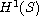Electron. J. Differential Equations, Vol. 2018 (2018), No. 53, pp. 1-6.

### Almost entire solutions of the Burgers equation Nicholas D. Alikakos, Dimitrios Gazoulis

Abstract:
We consider Burgers equation on the whole x-t plane. We require the solution to be classical everywhere, except possibly over a closed set S of potential singularities, which is
(a) a subset of a countable union of ordered graphs of differentiable functions,
(b) has one dimensional Hausdorff measure,, equal to zero.
We establish that under these conditions the solution is identically equal to a constant.

Submitted November 21, 2016. Published February 20, 2018.
Math Subject Classifications: 35B08, 35L65.
Key Words: Burgers equation; entropy solution; rigidity.

Show me the PDF file (180 KB), TEX file for this article.

 Nicholas D. Alikakos Department of Mathematics, University of Athens panepistimioupolis 15784 Athens, Greece email: nalikako@math.uoa.gr Dimitrios Gazoulis Department of Mathematics, University of Athens panepistimioupolis 15784 Athens, Greece email: dgazoulis@math.uoa.gr